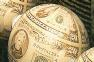Financial Calculus contentsFinancial Calculus
An introduction to derivative pricing
Contents

These are the contents as they will appear in the published version.

• Preface
• The Parable of the bookmaker
• Introduction
1. Expectation pricing
2. Arbitrage pricing
3. Expectation vs arbitrage
• Discrete processes
1. The binomial branch model
2. The binomial tree model
3. Binomial representation theorem
4. Overture to continuous models
• Continuous processes
1. Continuous processes
2. Stochastic calculus
3. Itôum; calculus
4. Change of measure - the C-M-G theorem
5. Martingale representation theorem
6. Constructing strategies
7. Black-Scholes model
8. Black-Scholes in action
• Pricing market securities
1. Foreign exchange
2. Equities and dividends
3. Bonds
4. Market price of risk
5. Quantos
• Interest rates
1. The interest rate market
2. A simple model
3. Single-factor HJM
4. Short-rate models
5. Multi-factor HJM
6. Interest rate products
7. Multi-factor models
• Bigger Models
1. General stock model
2. Log-normal models
3. Multiple stock models
4. Numeraires
5. Foreign currency interest-rate models
6. Arbitrage-free complete models
• Appendices